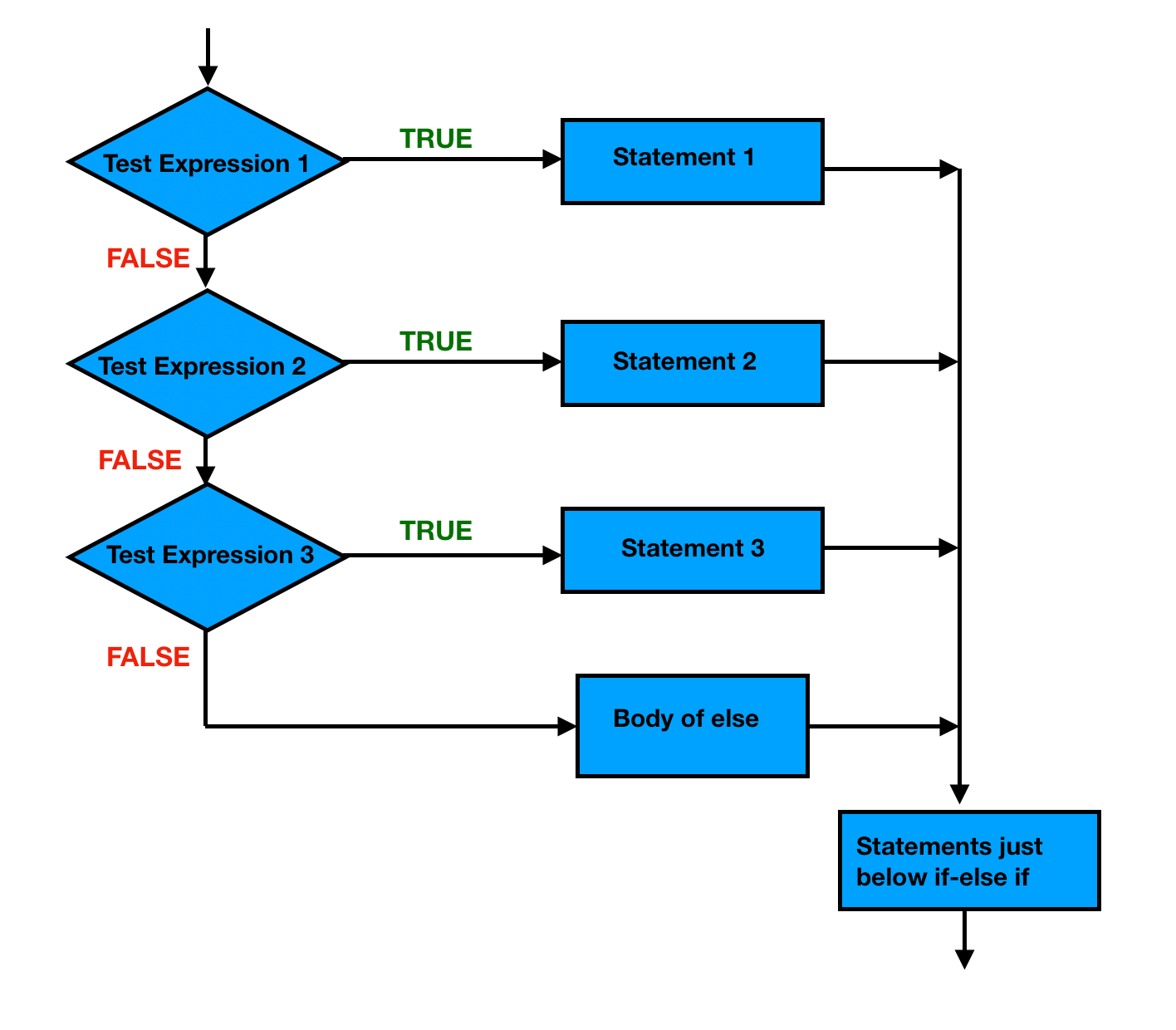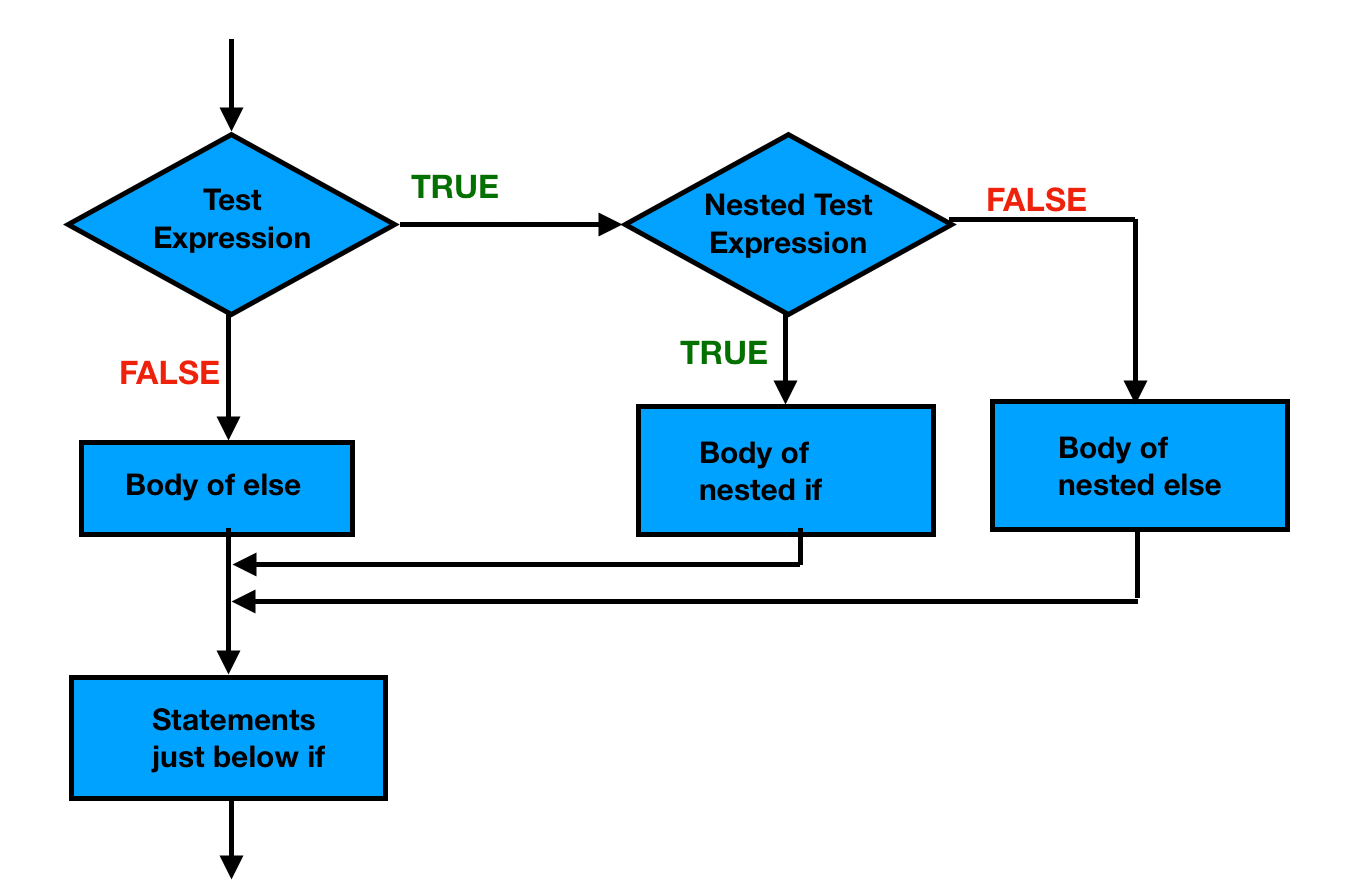# Kotlin if Expression

In this article, you will learn to use if expression in Kotlin with the help of examples.

There are different types of if expression in Kotlin:

• if-else expression
• nested if expression

#### if statement

The if statement checks the given condition. If the condition evaluates to be true then the block of code/statements will execute otherwise not.

Syntax :

```if (condition) {
// block of code to be executed if the condition is true
}```

#### if-else statement

The if statement executes a certain section of code if the test expression is evaluated to true. The if statement may have an optional else block. Statements inside the body of else statement are executed if the test expression is evaluated to false.

Syntax :

```if (condition) {
// block of code to be executed if the condition is true
} else {
// block of code to be executed if the condition is false
}```

### Kotlin if-else Expression

In Kotlin, if is an expression, i.e. it returns a value. But it is not used as standalone, it is used with if-else expression and the result of an if-else expression is assigned into a variable.

#### Flowchart:#### Example

```fun main(args: Array<String>) {
val num1 = 10
val num2 = 20
val result = if (num1 > num2) {
"\$num1 is greater than \$num2"
} else {
"\$num1 is smaller than \$num2"
}
println(result)
}```

#### Output

`10 is smaller than 20`

#### Example explained

In the above example, when x=10, y=20:

• condition 10>20 is checked, yields false and “10 is smaller than 20” gets printed.

when x=20, y=10 :

• condition 20>10 is checked, yields true and “10 is greater than 20” gets printed.

We can remove the curly braces of if-else body by writing if expression in only one statement.

For example:

```fun main(args: Array<String>) {
val num1 = 10
val num2 = 20
val result = if (num1 > num2) "\$num1 is greater than \$num2" else "\$num1 is smaller than \$num2"
println(result)
}```

Using if-else expression in one single line statement is like ternary operator in Java. Kotlin does not support any ternary operator.

When we want to execute a particular block of code among many blocks we can use if-else-if ladder.

Note: If the block of if, else, else-if contains more than one expression, the last expression is returned as the value of the block.

#### Flowchart:#### Example

```fun main(args: Array<String>) {
val marks = 65

val result = if (marks >= 80) {
print("You got A+ grade with marks: ")
marks
} else if (marks >= 75) {
print("You got A grade with marks: ")
marks
} else if (marks >= 60) {
print("You got B grade with marks: ")
marks
} else if (marks >= 45) {
print("You got C grade with marks: ")
marks
} else if (marks >= 35) {
print("You got D grade with marks: ")
marks
} else {
print("You are fail with marks: ")
marks
}
println(result)
}```

#### Output

`You got B grade with marks: 65`

#### Example explained

In the above example, when marks is 65:

• condition 1 is checked. 65>=80, yields false.
• condition 2 is checked. 65>=75, yields false.
• condition 3 is checked. 65>=60, yields true and “You got B grade with marks: 65” gets printed.

### Kotlin Nested if Expression

An if expression can be used inside the block of another if expression known as nested if expression.

#### Flowchart:#### Example

```fun main(args: Array<String>) {
val num1 = 10
val num2 = 20
val num3 = 30

val max = if (num1 > num2) {
if (num1 > num3)
num1
else
num3
} else {
if (num2 > num3)
num2
else
num3
}
println("maximum number = \$max")
}```

#### Output

`maximum number = 30`

#### Example explained

In the above example, when num1=10, num2=20, num3=30 :

• condition 10>20 is checked, yields false, skip if block and move to else block.
• condition 20>30 is checked, yields false.
• move to else part and return num3 as the maximum number.

when num1=30, num2=20, num3=10 :

• condition 30>20 is checked, yields true.
• condition 30>10 is checked, yields true, return num1 as the maximum number.